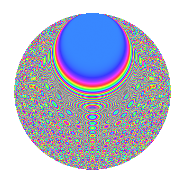# Properties

 Label 672.2.cjLevel 672 Weight 2 Character orbit cj Rep. character $$\chi_{672}(37,\cdot)$$ Character field $$\Q(\zeta_{24})$$ Dimension 512 Newform subspaces 1 Sturm bound 256 Trace bound 0

# Related objects

## Defining parameters

 Level: $$N$$ = $$672 = 2^{5} \cdot 3 \cdot 7$$ Weight: $$k$$ = $$2$$ Character orbit: $$[\chi]$$ = 672.cj (of order $$24$$ and degree $$8$$) Character conductor: $$\operatorname{cond}(\chi)$$ = $$224$$ Character field: $$\Q(\zeta_{24})$$ Newform subspaces: $$1$$ Sturm bound: $$256$$ Trace bound: $$0$$

## Dimensions

The following table gives the dimensions of various subspaces of $$M_{2}(672, [\chi])$$.

Total New Old
Modular forms 1056 512 544
Cusp forms 992 512 480
Eisenstein series 64 0 64

## Trace form

 $$512q + O(q^{10})$$ $$512q - 64q^{14} + 8q^{16} + 8q^{18} + 64q^{20} + 16q^{22} + 16q^{23} - 40q^{28} + 96q^{31} + 48q^{35} - 80q^{38} + 64q^{40} - 32q^{43} - 8q^{44} - 48q^{50} + 24q^{52} + 32q^{53} - 32q^{58} + 64q^{59} - 48q^{60} - 48q^{64} + 48q^{66} - 16q^{67} + 72q^{70} - 128q^{71} - 144q^{74} - 48q^{78} + 24q^{80} + 40q^{82} - 88q^{88} + 48q^{91} + 128q^{92} - 24q^{94} - 40q^{96} - 88q^{98} + O(q^{100})$$

## Decomposition of $$S_{2}^{\mathrm{new}}(672, [\chi])$$ into newform subspaces

Label Dim. $$A$$ Field CM Traces $q$-expansion
$$a_2$$ $$a_3$$ $$a_5$$ $$a_7$$
672.2.cj.a $$512$$ $$5.366$$ None $$0$$ $$0$$ $$0$$ $$0$$

## Decomposition of $$S_{2}^{\mathrm{old}}(672, [\chi])$$ into lower level spaces

$$S_{2}^{\mathrm{old}}(672, [\chi]) \cong$$ $$S_{2}^{\mathrm{new}}(224, [\chi])$$$$^{\oplus 2}$$

## Hecke Characteristic Polynomials

There are no characteristic polynomials of Hecke operators in the database